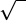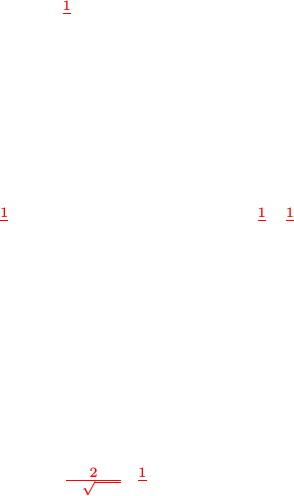Study Guides (400,000)
US (230,000)
UC-Irvine (3,000)
CHEM (100)
Quiz

# CHEM 132B Study Guide - Quiz Guide: Spherical Harmonics, Position Operator, Electron Rest MassExam

Department
Chemistry
Course Code
CHEM 132B
Professor
Eric Potma
Study Guide
Quiz

This preview shows pages 1-2. to view the full 7 pages of the document.Practice Final Key
Version A
Problem 1
Consider the orthonormal set |pi, which are eigenfunctions of the linear momen-
tum operator bp. State whether the following are true or false.
a) The states |piare eigenstates of the Hamiltonian b
H(x) with b
V(x) = 0.
True
b) The states |piare eigenstates of the position operator bx.False
c) hp|bp2|pi= 0 if p6=p.True
d) hp|[bx, bp]|pi= 0. False
Problem 2
Consider the spherical harmonic functions Yl,ml(θ, φ). State whether the follow-
ing are true or false.
a) The magnitude of the angular momentum of an orbital with Y3,2is 2h.
True
b) There are 2l+ 1 nodes in φfor each l.False
c) The function Y1,1(θ, φ) is orthogonal to all other spherical harmonic
functions. True
d) The probability distribution of ﬁnding the electron within a given surface
area Aon the sphere (θ, φ) is P(r) = r2|Rn,l|2.False
Problem 3
Molecular bond vibrations can be described well as quantum harmonic oscilla-
tors. Assuming an atom A and an atom B, the eﬀective mass of the oscillator
is given by µ=mAmB/(mA+mB). Assume the spring constant of diﬀerent
molecular bonds to be roughly the same. State whether the following are true
or false.
a) The excitation energy of the v= 0 to v= 1 transition for the C-C bond
vibration is higher than for the C-H bond vibration. False
b) The excitation energy of the v= 1 to v= 2 transition for the O2bond
vibration is higher than for the N2bond vibration. False
c) The mean square extension of the O2bond vibration in the v= 0 state is
smaller than in the v= 10 state. True
d) The average extension of the N2bond vibration is larger in the v= 0 state
than in the v= 10 state. False

Only pages 1-2 are available for preview. Some parts have been intentionally blurred.Problem 4
Consider the hydrogen atom. The atom is initially in the 2s1conﬁguration.
a) Give the term symbol(s) of the atom in this conﬁguration.
L= 0, S=1
2, so 2S+ 1 = 2
2S1/2
b) Consider the total angular momentum of the electron in this hydrogen
atom. What are the projections along zof its total angular momentum?
J=1
2, so projections are MJ=1
2,1
2
c) The electron is excited to the n= 3 state. A measurement reveals that the
magnetic quantum number (ml) of the state is 0. Give a full expression
of the wavefunction Ψ(r, θ, φ) of the excited state.
The electron is the 3p state with n= 3, l= 1 and ml= 0:
Ψ(r, θ, φ) = 2
8110π1
a7/2r2er/3acos θ
d) Give the term symbols of the excited state. Indicate which state lies low-
est in energy.
2P3/2and 2P1/2, with 2P1/2lowest in energy.